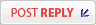View unanswered posts | View active topics It is currently Mon Jul 22, 2019 9:58 amPage 1 of 1 [ 4 posts ]
 Print view Previous topic | Next topic
Generating Code for Arrays
Author Message

Joined: Sat Feb 02, 2013 9:40 am
Posts: 910An epiphany moment. I just realized that one needs to perform double precision arithmetic while calculating code for handling array indexes because ratios between array indexes of less than one are possible. (The code finally got a divide by zero error while handling arrays – it was processing a ratio of 2/8 using integers). The array sizes are always evenly divisible by one another so the ratios can work out to things like ¼ or 1/8. One starts with the total cumulative size of the array then divides it down by the product of all the next sizes. The resulting output code is a series of multiplies and adds.
It’s quite complex.
The greatest number of array indexes I’ve ever used is about six or seven. It was for quite a complex financial calculation where a large number of constraints were parameterized and controllable by the user. A close second was five dimensional arrays for handling a game’s graphics.

_________________
Robert Finch http://www.finitron.ca

Tue Nov 08, 2016 4:15 pmJoined: Wed Jan 09, 2013 6:54 pm
Posts: 1206I'm baffled and intrigued! Could you perhaps say more? When might one be taking ratios of array indices?

Tue Nov 08, 2016 5:10 pmJoined: Sat Feb 02, 2013 9:40 am
Posts: 910I got to wondering why the code was so complicated and realized it was coded in a backwards fashion. It worked but sheez, it could be better. I had a look at the code for a couple of other compilers and ended up re-writing it to be much simpler. No need for all the fancy factoring and division. Part of the problem now might be that it uses more registers. The way I had it written I think IIRC it interspersed multiplies and adds so registers could be reused. The way it is now it does a whole bunch of multiplies placing the results in different registers, then does a whole bunch of adds.

The way it was written, it calculated the amount to multiply by taking the total size and dividing it by the product of all the sizes that made up the type. It's difficult to explain so I'll post some of the code.

This bit of code determined the values of the all indicies by parsing the expressions inside the []. It collected up all the expressions into expression nodes (rnode). A thing to note is it found the total size of the array at that point in the indexing and stored it in a size array sz[].
Code:
for (na = 0; na < 20 && p; na++) {
sz[na] = p->size;
if (lastst== openbr) {
NextToken();
expression(&rnode[na]);
if (rnode[na]==nullptr) {
dfs.printf("rnode[] is null\n");
goto j1;
}
needpunc(closebr,9);
}
else {
rnode[na] = makeinode(en_icon, 0);
}
p = p->GetBtp();
}
na--;
if (na>19) {
error(ERR_TOOMANYDIMEN);

This bit of code then took the sizes determined above based on the indicies, and created multiply nodes that ultimately resulted in the generation of code. Note it has to calculate multiplication factors by dividing one size by the subsequent one.
Code:
default:
fact = sz[na];
for (ii = 1,nn = na-1; nn >= 0; nn--, ii++) {
fact[ii] = sz[nn]/sz[nn+1];
}
for (ii = 0; ii < na; ii++) {
cnode[ii] = makeinode(en_icon,size/MultiplyFact(ii+1,fact));  // MultiplyFact multiples all the factors together
cnode[ii]->constflag = TRUE;
cnode[ii]->isUnsigned = tp1->isUnsigned;
qnode[ii] = makenode(en_mulu,rnode[ii],cnode[ii]);
qnode[ii]->constflag = rnode[ii]->constflag;
}
cnode[ii] = makeinode(en_icon,fact);
cnode[ii]->constflag = TRUE;
cnode[ii]->isUnsigned = tp1->isUnsigned;
qnode[ii] = makenode(en_mulu,rnode[ii],cnode[ii]);
qnode[ii]->constflag = rnode[ii]->constflag;

Code:
for (ii = 1; ii <= na; ii++) {
ep2->constflag = ep2->p->constflag && qnode[ii]->constflag;
ep2->isUnsigned = ep2->p->isUnsigned && qnode[ii]->isUnsigned;
}

_________________
Robert Finch http://www.finitron.ca

Tue Nov 08, 2016 9:05 pmJoined: Wed Jan 09, 2013 6:54 pm
Posts: 1206Thanks - I'm dimly getting a picture here, that you're constructing code to access multidimensional arrays, so there will need to be some multiplying. Somehow you'd arranging things so that you'd formed a large product and were then dividing into it. That kind of makes sense to me - I'm not sure how you'd wind up with fractions, so long as you always divide by a divisor. But overall, if you now feel you'd taken a wrong turning, trying to understand how you got there is perhaps not the best way forward!

Wed Nov 09, 2016 9:49 am
Display posts from previous:  Sort byPage 1 of 1 [ 4 posts ]

#### Who is online

Users browsing this forum: No registered users and 1 guest

 You cannot post new topics in this forumYou cannot reply to topics in this forumYou cannot edit your posts in this forumYou cannot delete your posts in this forumYou cannot post attachments in this forum

Search for:
 Jump to:  Select a forum ------------------ General Discussions Newbies Software    General programming    Languages and tools    Kernels and operating systems Hardware    Hardware in general    CPU/MCU choices and designs    Implementation and Construction Programmable logic Simulation and emulation Nostalgia Projects Anycpu.org